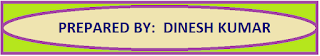### Common Errors in Secondary Mathematics

Common Errors Committed  by the  Students  in Secondary Mathematics   Errors  that students often make in doing secondary mathematics  during their practice and during the examinations  and their remedial measures are well explained here stp by step.  Some Common Errors in Mathematics

# CBSE Assignments class 12 Mathematics

Chapter-wise mathematics assignment for class 12. Important and useful extra questions strictly according to the CBSE syllabus and pattern with answer key

CBSE Mathematics is a very good platform for the students and is contain the assignments for the students from 9th to 12th standard.

Here students can find very useful content which is very helpful to handle final examinations effectively.

For better understanding of the topic students should revise NCERT book with all examples and then start solving the chapter-wise assignments.

These assignments cover all the topics and are strictly according to the CBSE syllabus.

With the help of these assignments students can easily achieve the examination level and  can reach at the maximum height.

 Class 12Mathematics  Assignment Case Study Based QuestionChapter 1: Mathematics Assignment on Relations & FunctionsChapter 3: Mathematics Assignment on MatricesChapter 3: Chapter 3 Sol. of Main QuestionsChapter 4: Mathematics Assignment on DeterminantsChapter 7: Mathematics Assignment on IntegrationChapter 8: Mathematics Assignment on Applications of IntegralChapter 10: Mathematics Assignment on  Vector AlgebraChapter 12: Mathematics Assignment on Linear Programming ProblemsChapter 13: Mathematics Assignment on Probability

##CBSE Mathematics also contains chapter-wise maths formulas /basic points / definitions / lesson plans useful for the teachers and many other blogs which are useful for all maths loving people.

A platform for the mathematics student is provided where students can fulfill all their study related needs. So click on the link below to enter the RESOURCE CENTRE.